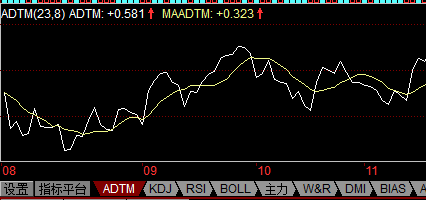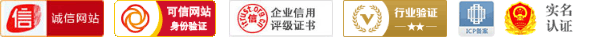DTM:=IF(OPEN<=REF(OPEN,1),0,MAX((HIGH-OPEN),(OPEN-REF(OPEN,1))));
DBM:=IF(OPEN>=REF(OPEN,1),0,MAX((OPEN-LOW),(OPEN-REF(OPEN,1))));
STM:=SUM(DTM,N);
SBM:=SUM(DBM,N);

1.如果开盘价≤昨日开盘价，DTM=0

2.如果开盘价≥昨日开盘价，DBM=0

3.STM=DTM在N日内的和
4.SBM=DBM在N日内的和

7.参数N设置为23日，参数M设置为8日